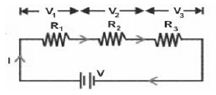Physics

# Equivalent Resistance and its Uses in Circuit

Sometimes several resistances are connected together for different purposes. A connection of more than one resistance together is called a combination of resistances.

Equivalent resistance: If a single resistance is used instead of a combination of resistance and if the current and potential difference is not changed in the circuit. then that resistance is called the equivalent resistance of the combination.

Combination of resistances is of two types, e.g. – series combination and parallel combination.

Series combination of resistances

Figure shows resistors R1, R2 and R3 are connected in series. The resistances are connected one after another successively. In this case, the same current I is flowing through each of the resistors. Now we shall calculate the equivalent resistance of these three resistances those are connected in series.From Ohm’s law we get,

The potential difference across resistance R1, V1 = IR1,

The potential difference across resistance R2, V2 = IR2

The potential difference across resistance R3, V3 = IR3

If V is the potential difference between the two terminals of all the resistors, i.e. the potential difference across the combination,

V = V1 + V2 + V3 = IR1 + IR2 + IR3 = I (R1 + R2 + R3)

Now if three resistances R1, R2 and R3 are replaced by a single resistance RS, so that same current I flows through the circuit and the potential difference V across them remains unchanged, then RS is the equivalent resistance of the combination. In case of equivalent resistance, V = IRS.

Therefore, the equivalent resistance of resistors connected is series is equal to the sum of the different resistances included in the combination. The value of the equivalent resistance in series combination is greater than that of individual resistances.